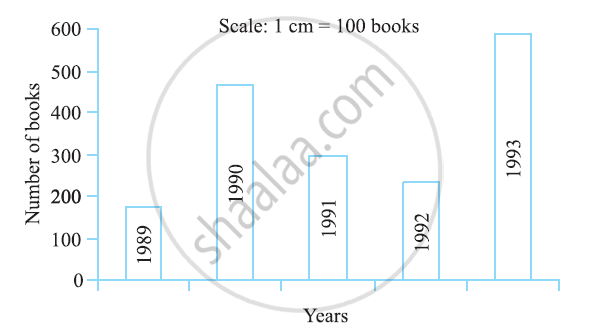# Read the Bar Graph (See the Given Figure) Which Shows the Number of Books Sold by a Bookstore During Five Consecutive Years and Answer the Questions that Follow: - Mathematics

Read the bar graph (see the given figure) which shows the number of books sold by a bookstore during five consecutive years and answer the questions that follow:1) About how many books were sold in 1989? 1990? 1992?

2) In which year were about 475 books sold? About 225 books sold?

3) In which years were fewer than 250 books sold?

4) Can you explain how you would estimate the number of books sold in 1989?

#### Solution

1) In 1989, 175 books were sold. In 1990, 475 books were sold. In 1992, 225 books were sold.

2) From the graph, it can be concluded that 475 books were sold in the year 1990 and 225 books were sold in the year 1992.

3) From the graph, it can be concluded that in the years 1989 and 1992, the number of books sold were less than 250.

4) From the graph, it can be concluded that the number of books sold in the year 1989 is about 1 and 3/4 th part of 1 cm.

We know that the scale is taken as 1 cm = 100 books.

100 + 3/4 xx 100 = 100 + 75 = 175

Therefore, about 175 books were sold in the year 1989.

Concept: Concept of Bar Graph
Is there an error in this question or solution?

#### APPEARS IN

NCERT Class 7 Maths
Chapter 3 Data Handling
Exercise 3.3 | Q 2 | Page 72